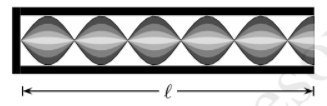# Problem: Consider another organ pipe which has one end open and one end closed. Determine the wave length of the sound wave in this hollow pipe. 1. λ = 4ℓ/13 2. λ = 4ℓ/11 3. λ = 4ℓ/5 4. λ = 4ℓ/3 5. λ = 4ℓ 6. λ = 4ℓ/7 7. λ = 4ℓ/15 8. λ = 4ℓ/17 9. λ = 4ℓ/9

###### Problem Details

Consider another organ pipe which has one end open and one end closed. Determine the wave length of the sound wave in this hollow pipe.

1. λ = 4ℓ/13

2. λ = 4ℓ/11

3. λ = 4ℓ/5

4. λ = 4ℓ/3

5. λ = 4ℓ

6. λ = 4ℓ/7

7. λ = 4ℓ/15

8. λ = 4ℓ/17

9. λ = 4ℓ/9